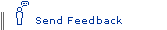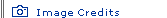All the Grains of Sand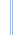Infinite Secrets homepage

For ages 12 and older.

How many grains of sand would it take to fill the universe? This is a problem that the Greek mathematician Archimedes tried to solve in the third century B.C. In this activity, you get to try Archimedes' problem (on a slightly smaller scale) by estimating the number of grains of sand it would take to fill different containers.

You will need

• metric ruler or measuring tape
• several regular-shaped containers, such as square or rectangular boxes, or straight-sided cylindrical glasses or cans
• calculator
• paper
• pencil

What to do

1. Select a container. If the container is a square or rectangle, use the ruler or tape measure to find the length, height, and width of the container in centimeters. If the container is a cylinder (such as a straight-sided glass or can), measure the height of the container and the diameter of the container's base in centimeters. The diameter is the length across the widest part of the circular base. Record your measurements on a separate piece of paper.

2. Use the measurements you recorded to calculate the volume of the container. The volume of an object is the amount of space the object occupies. The following are the formulae for the volumes of some regular containers, where l is the length, w is the width, h is the height, and r is the radius, which is 1/2 of the diameter. Assume that pi (π) is equal to 3.14.

• Volume of a Cube = l x w x h
• Volume of a Rectangle = l x w x h
• Volume of a Cylinder = πr2h

3. Assume that a grain of sand is basically a sphere, with a radius of 0.025 cm. The volume of a sphere can be found using the formula Volume of a Sphere = 4/3 πr3 Calculate the volume of a grain of sand. Record your calculation.

4. Find the approximate number of grains of sand that it would take to fill the container by dividing the volume of the container by the volume of a grain of sand:
number of grains of sand in container = container volume/sand grain volume

5. Repeat the process, using different containers. Which container holds the most sand? Which holds the least?

6. The volume of Earth is about 1.083 x 1027 cm3. How many grains of sand would it take to fill Earth?Learning MoreFilling & Wrapping: Three-Dimensional Measurement
Lappan, Glenda, et. al. Needham, MA: Prentice Hall, 2002.
Provides seven investigations that explore the surface area and volume of objects such as rectangular prisms, cylinders, cones, and spheres. For young adults.

The Sand Reckoner
Describes how Archimedes tried to estimate the number of grains of sand it would take to fill the universe, in both Archimedes' words and modern-day language. For young adults and adults.Powers of TenTo estimate the number of grains of sand it would take to fill the universe, Archimedes had to create a way to express very large numbers. Today, scientists and mathematicians use scientific notation to handle such numbers. In scientific notation, a number is expressed as a number between 1 and 10 and then multiplied by a power of 10. The number of the exponent is equal to the number of zeros added on the end. For example, 1,000,000 can be written as 1x106 (which is read as "one times ten to the sixth"), and 1,000,000,000 is 1x109. In today's notation, Archimedes' estimate for the number of grains of sand that it would take to fill the then-known universe was 1 x 1063 grains of sand!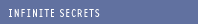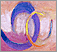Contemplating Infinity Philosophically, the concept remains a mind-bender.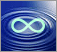Working with Infinity Mathematicians have become increasingly comfortable with the concept.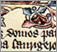Great Surviving Manuscripts Ancient documents offer a tantalizing glimpse of lost cultures.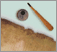The Archimedes Palimpsest Follow the 1,000-year-long journey of the Archimedes manuscript.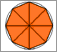Approximating Pi See Archimedes' geometrical approach to estimating pi.